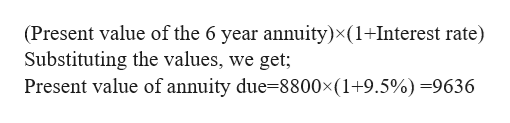# If the present value of an ordinary, 6-year annuity is \$8,800 and interest rates are 9.5 percent, what’s the present value of the same annuity due? (Round your answer to 2 decimal places.)PV = \$_______.__

Question
89 views

If the present value of an ordinary, 6-year annuity is \$8,800 and interest rates are 9.5 percent, what’s the present value of the same annuity due? (Round your answer to 2 decimal places.)

PV = \$_______.__

check_circle

Step 1

An annuity due is a type of annuity in which payments are made at regular time interval and at the beginning of every period.

Step 2

The present value of the same annuity due is calculated as:

Step 3help_outlineImage Transcriptionclose(Present value of the 6 year annuity)x(1+Interest rate) Substituting the values, we get; Present value of annuity due-8800x(1+9.5%) =9636 fullscreen

### Want to see the full answer?

See Solution

#### Want to see this answer and more?

Solutions are written by subject experts who are available 24/7. Questions are typically answered within 1 hour.*

See Solution
*Response times may vary by subject and question.
Tagged in